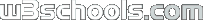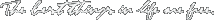Search W3Schools :
 ADVERTISEMENTS XML Certification Download XML editor Custom Programming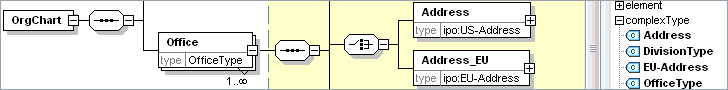JS Basic
JS HOME
JS Introduction
JS How To
JS Where To
JS Statements
JS Variables
JS Operators
JS Comparisons
JS If...Else
JS Switch
JS Popup Boxes
JS Functions
JS For Loop
JS While Loop
JS Break Loops
JS For...In
JS Events
JS Try...Catch
JS Throw
JS onerror
JS Special Text
JS Guidelines

JS Objects
JS Objects Intro
JS String
JS Date
JS Array
JS Boolean
JS Math
JS RegExp
JS HTML DOM

JS Browser
JS Validation
JS Animation
JS Image Maps
JS Timing
JS Create Object
JS Summary

Examples/Quiz
JS Examples
JS Object Examples
JS DOM Examples
JS Quiz
JS Exam

JS References
JS Objects
JS HTML DOM

# JavaScript Math Object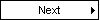The Math object allows you to perform mathematical tasks.

## Examples

round()
How to use round().

random()
How to use random() to return a random number between 0 and 1.

max()
How to use max() to return the number with the highest value of two specified numbers.

min()
How to use min() to return the number with the lowest value of two specified numbers.

## Complete Math Object Reference

For a complete reference of all the properties and methods that can be used with the Math object, go to our complete Math object reference.

The reference contains a brief description and examples of use for each property and method!

## Math Object

The Math object allows you to perform mathematical tasks.

The Math object includes several mathematical constants and methods.

Syntax for using properties/methods of Math:

 ```var pi_value=Math.PI; var sqrt_value=Math.sqrt(16);```

Note: Math is not a constructor. All properties and methods of Math can be called by using Math as an object without creating it.

## Mathematical Constants

JavaScript provides eight mathematical constants that can be accessed from the Math object. These are: E, PI, square root of 2, square root of 1/2, natural log of 2, natural log of 10, base-2 log of E, and base-10 log of E.

You may reference these constants from your JavaScript like this:

 ```Math.E Math.PI Math.SQRT2 Math.SQRT1_2 Math.LN2 Math.LN10 Math.LOG2E Math.LOG10E```

## Mathematical Methods

In addition to the mathematical constants that can be accessed from the Math object there are also several methods available.

The following example uses the round() method of the Math object to round a number to the nearest integer:

 `document.write(Math.round(4.7));`

The code above will result in the following output:

 `5`

The following example uses the random() method of the Math object to return a random number between 0 and 1:

 `document.write(Math.random());`

The code above can result in the following output:

 ``` document.write(Math.random()) ```

The following example uses the floor() and random() methods of the Math object to return a random number between 0 and 10:

 `document.write(Math.floor(Math.random()*11));`

The code above can result in the following output:

 ``` document.write(Math.floor(Math.random()*11)) ```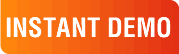Ektron CMS400.NET Version 7.6 delivers all of the flexibility and features you need to deploy the Web site you want, quickly and efficiently. learn more...

6 ways to take your site to the next level with Ektron: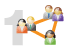Create site stickiness through social networking. Keep it personal, relevant and interactive and they'll come back for more.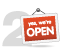Keep it open. Your site needs to be ready and able to connect to outside services. Ektron's open API gives you maximum flexibility.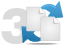Streamline content and document management. Users need to quickly and intuitively find and add information.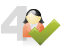Empower your content authors. Reduce IT bottlenecks by allowing business users to create and edit Web content and forms.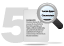Climb to the top of search rankings. SEO tools, URL aliasing and eCommerce for your digital marketing strategy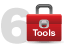Add powerful Web 2.0 tools like blogs, wikis, forums, geo-mapping, rating systems and RSS feeds easily.
 See why there are 20,000+ Ektron integrations worldwide. Request an INSTANT DEMO or download a FREE TRIAL today.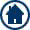HOME or Top of Page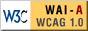Printer FriendlyW3Schools is for training only. We do not warrant the correctness of its content. The risk from using it lies entirely with the user. While using this site, you agree to have read and accepted our terms of use and privacy policy. Copyright 1999-2009 by Refsnes Data. All Rights Reserved.